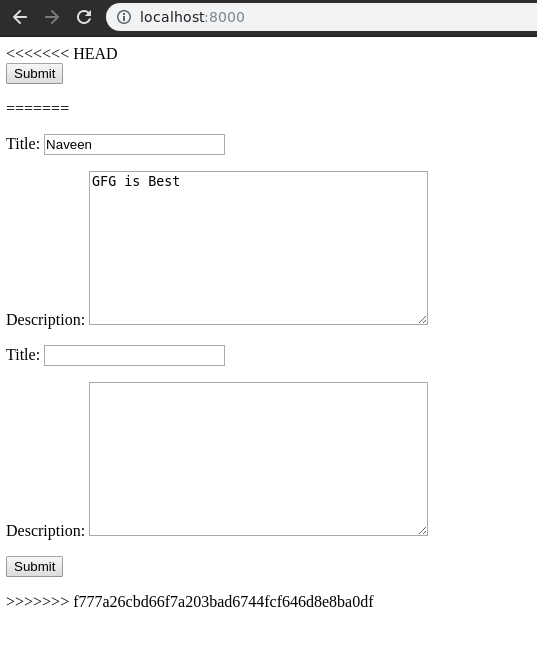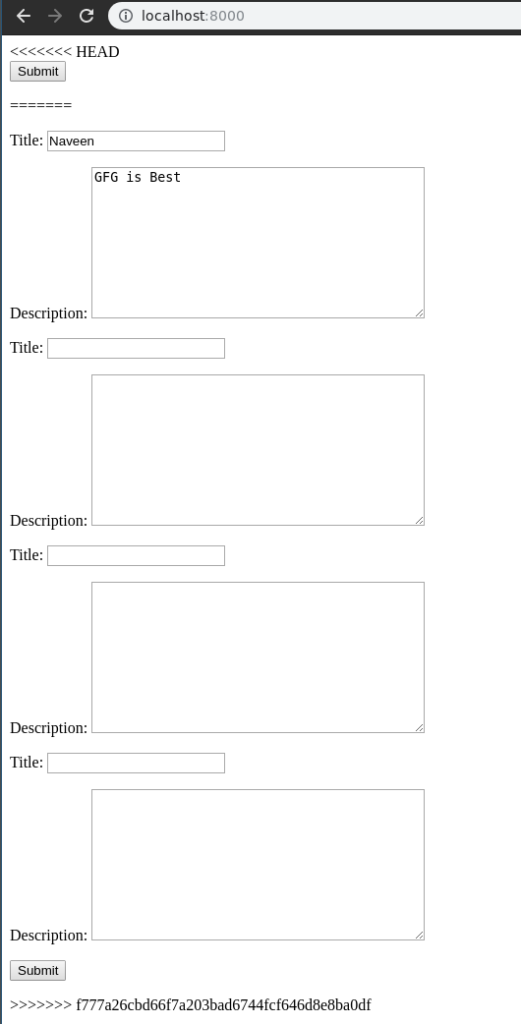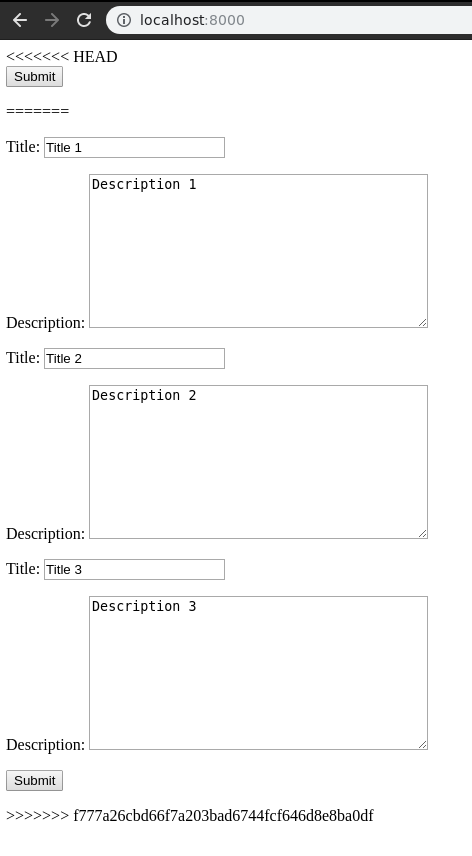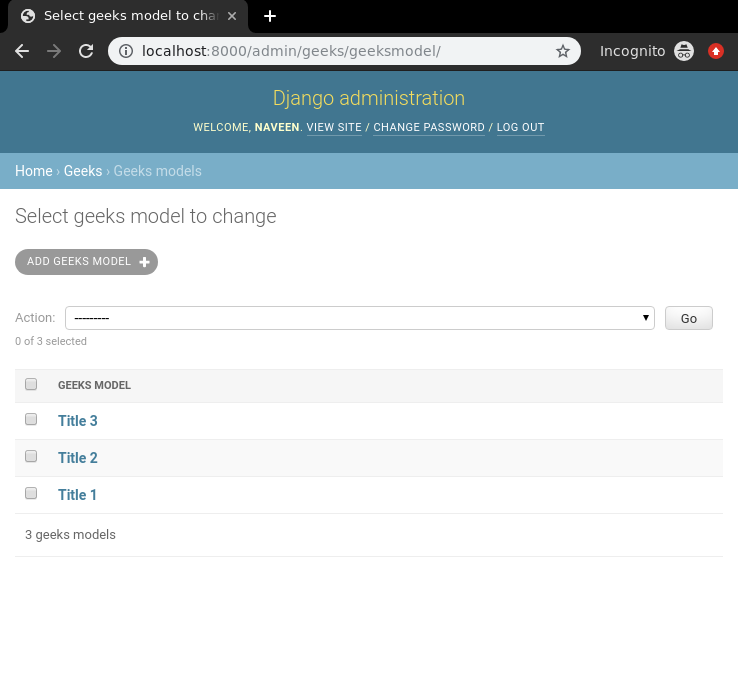# Django ModelFormSets

• Difficulty Level : Basic
• Last Updated : 09 Jan, 2020

ModelFormsets in a Django is an advanced way of handling multiple forms created using a model and use them to create model instances. In other words, ModelFormsets are a group of forms in Django. One might want to initialize multiple forms on a single page all of which may involve multiple POST requests, for example

```class GeeksModel(models.Model):
title = models.CharField(max_length = 200)
description = models.TextField()
```

Now if one wants to create a modelformset for this model, modelformset_factory needs to be used. A formset is a layer of abstraction to work with multiple forms on the same page. It can be best compared to a data grid.

```from django.forms import formset_factory
GeeksFormSet = modelformset_factory(GeeksModel)
```

### Creating and using Django ModelFormsets

Illustration of Rendering Django ModelFormsets manually using an Example. Consider a project named `geeksforgeeks` having an app named `geeks`.

Refer to the following articles to check how to create a project and an app in Django.

In your geeks app make a new file called models.py where you would be making all your models. To create a Django model you need to use Django Models. Let’s demonstrate how,
In your` models.py` Enter the following,

 `# import the standard Django Model ` `# from built-in library ` `from` `django.db ``import` `models ` `  `  `# declare a new model with a name "GeeksModel" ` `class` `GeeksModel(models.Model): ` ` `  `    ``# fields of the model ` `    ``title ``=` `models.CharField(max_length ``=` `200``) ` `    ``description ``=` `models.TextField() ` ` `  `    ``# renames the instances of the model ` `    ``# with their title name ` `    ``def` `__str__(``self``): ` `        ``return` `self``.title `

Let’s explain what exactly is happening, left side denotes the name of the field and to right of it, you define various functionalities of an input field correspondingly. A field’s syntax is denoted as
Syntax :

`Field_name = models.FieldType(attributes)`

Now to create a simple formset of this form, move to views.py and create a `formset_view` as below.

 `from` `django.shortcuts ``import` `render ` ` `  `# relative import of forms ` `from` `.forms ``import` `GeeksForm ` ` `  `# importing formset_factory ` `from` `django.forms ``import` `formset_factory ` ` `  `def` `formset_view(request): ` `    ``context ``=``{} ` ` `  `    ``# creating a formset ` `    ``GeeksFormSet ``=` `modelformset_factory(GeeksForm) ` `    ``formset ``=` `GeeksFormSet() ` `     `  `    ``# Add the formset to context dictionary ` `    ``context[``'formset'``]``=` `formset ` `    ``return` `render(request, ``"home.html"``, context) `

To render the formset through HTML, create a html file “home.html”. Now let’s edit `templates > home.html`

 `<``form` `method``=``"POST"` `enctype``=``"multipart/form-data"``> ` `    ``{% csrf_token %} ` `    ``{{ formset.as_p }} ` `    ``<``input` `type``=``"submit"` `value``=``"Submit"``> ` ` `

All set to check if our formset is working or not let’s visit http://localhost:8000/.Our `modelformset` is working completely. Let’s learn how to modify this formset to use extra features of this formset.

### How to create Multiple forms using Django ModelFormsets

Django formsets are used to handle multiple instances of a form. One can create multiple forms easily using `extra` attribute of Django Formsets. In geeks/views.py,

 `from` `django.shortcuts ``import` `render ` ` `  `# relative import of forms ` `from` `.models ``import` `GeeksModel ` ` `  `# importing formset_factory ` `from` `django.forms ``import` `modelformset_factory ` ` `  `def` `modelformset_view(request): ` `    ``context ``=``{} ` ` `  `    ``# creating a formset and 5 instances of GeeksForm ` `    ``GeeksFormSet ``=` `modelformset_factory(GeeksModel, fields ``=``[``'title'``, ``'description'``], extra ``=` `3``) ` `    ``formset ``=` `GeeksFormSet() ` ` `  `             `  `    ``# Add the formset to context dictionary ` `    ``context[``'formset'``]``=` `formset ` `    ``return` `render(request, ``"home.html"``, context) `

The keyword argument `extra` makes multiple copies of same form. If one wants to create 5 forms enter `extra = 5` and similarly for others. Visit http://localhost:8000/ to check if 5 forms are created.### Handling Multiple forms using Django Formsets

Creating a form is much easier than handling the data entered into those fields at the back end. Let’s try to demonstrate how one can easily use the data of a model formset in a view. When trying to handle formset, Django formsets required one extra argument {{ formset.management_data }}. To know more about Management data, Understanding the ManagementForm.
In `templates/home.html`,

 `<``form` `method``=``"POST"` `enctype``=``"multipart/form-data"``> ` `     `  `    ``` `    ``{{ formset.management_data }} ` ` `  `    ``` `    ``{% csrf_token %} ` ` `  `    ``` `    ``{{ formset.as_p }} ` `     `  `    ``<``input` `type``=``"submit"` `value``=``"Submit"``> ` ` `

Now to check how and what type of data is being rendered edit formset_view to print the data. In `geeks/view.py`,

 `from` `django.shortcuts ``import` `render ` ` `  `# relative import of forms ` `from` `.forms ``import` `GeeksForm ` ` `  `# importing formset_factory ` `from` `django.forms ``import` `formset_factory ` ` `  `def` `formset_view(request): ` `    ``context ``=``{} ` ` `  `    ``# creating a formset and 5 instances of GeeksForm ` `    ``GeeksFormSet ``=` `formset_factory(GeeksForm, extra ``=` `3``) ` `    ``formset ``=` `GeeksFormSet(request.POST ``or` `None``) ` `     `  `    ``# print formset data if it is valid ` `    ``if` `formset.is_valid(): ` `        ``for` `form ``in` `formset: ` `            ``print``(form.cleaned_data) ` `             `  `    ``# Add the formset to context dictionary ` `    ``context[``'formset'``]``=` `formset ` `    ``return` `render(request, ``"home.html"``, context) `

Now let’s try to enter data in the formset through http://localhost:8000/Hit submit and data will be saved in GeeksModel where server is running. One can use this data in any manner conveniently now.My Personal Notes arrow_drop_up
Recommended Articles
Page :